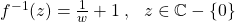Complex Functions: Domains, Image Sets and Inverses

I can imagine having to refer to these notes regularly, so I'm putting them here!

Image Sets

1. State the domain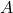of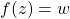.
2. Rearrange so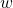is a function of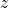(to discover the condition under whichremains valid.

e.g., for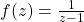: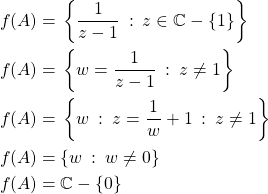Domain of Combined Functions

Domain of combined functions are the intersection (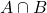) of the domains of all component functions and that of the combined function. e.g: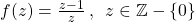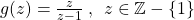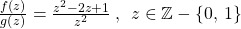Domain of Composite Functions

For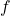and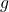with domainsand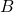respectively, the domain ofis: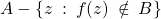e.g., for: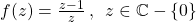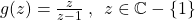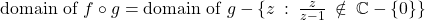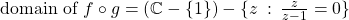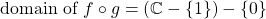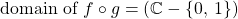Inverses

1. Determine image set of.
2. Invert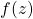to find a uniquein the domain of.

For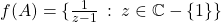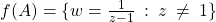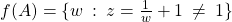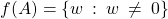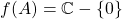(all same as above for finding an image set)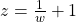gives a unique soluition in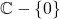, hencehas a unique inverse rule: